# 使用 MLlib 进行机器学习（十 - 下）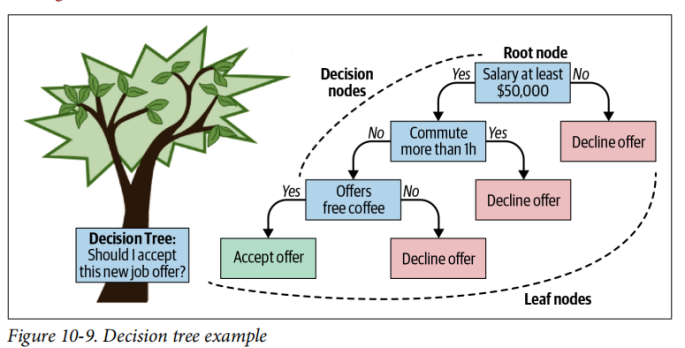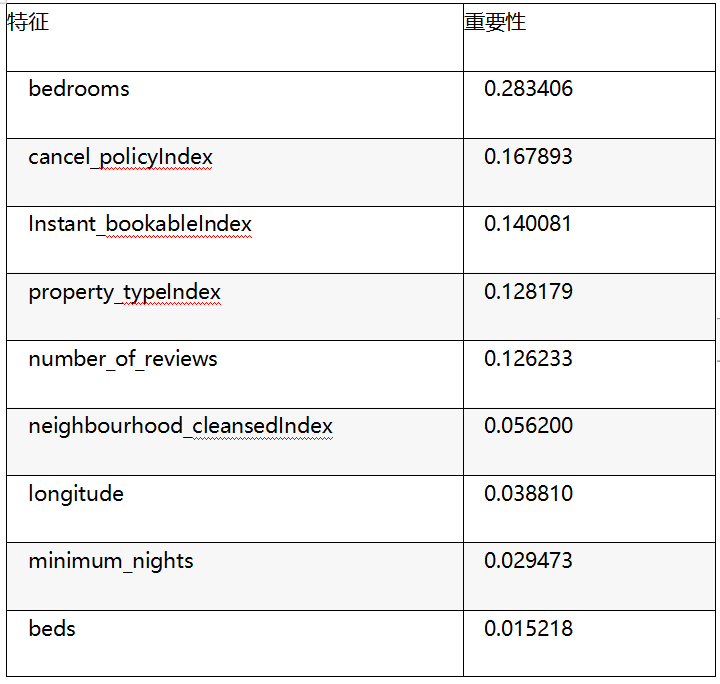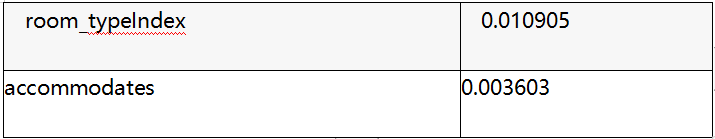bootstrapping 程序是一种通过从原始数据中进行可替换采样来生成新数据的技术。每个决策树都在数据集的不同 bootstrapping 样本上训练，生成的决策树略有不同，然后汇总它们的预测。这种技术称为 bootstrapping aggregating 或 bagging。在典型的随机森林实现中，每棵树都采样相同数量的数据点，并从原始数据集中进行替换，并且可以通过 subsamplingRate 参数控制该数量。

bagging 的主要缺点是树都是高度相关的，因此可以在数据中学习相似的模式。为了减轻这个问题，每次要进行拆分时，只考虑列的随机子集（RandomForestRegressor 只需要 1/3 特征以及提供√#features 给 RandomForestClassifier）。由于你引入了这种随机性，因此你通常希望每棵树都比较浅。你可能在想：这些树中的每一个都会比任何单个决策树表现更差，那么这种方法可能会更好吗？事实证明，每棵树都从你的数据集中学到了一些不同的东西，并将这些“弱”学习者集合成一个整体，使森林比单个决策树更健壮。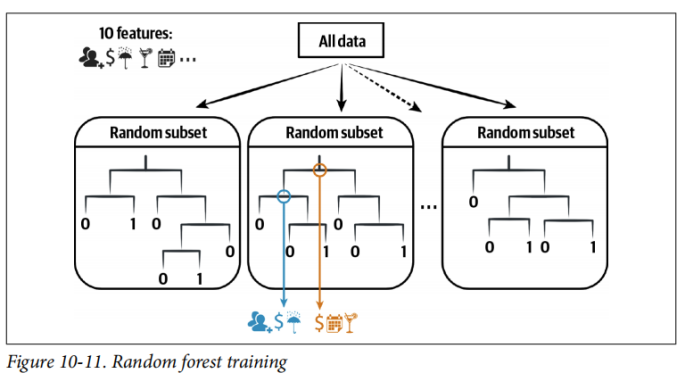In Python

from pyspark.ml.regression import RandomForestRegressor

rf = RandomForestRegressor(labelCol="price", maxBins=40, seed=42)

// In Scala

import org.apache.spark.ml.regression.RandomForestRegressor

val rf = new RandomForestRegressor()

.setLabelCol("price")

.setMaxBins(40)

.setSeed(42)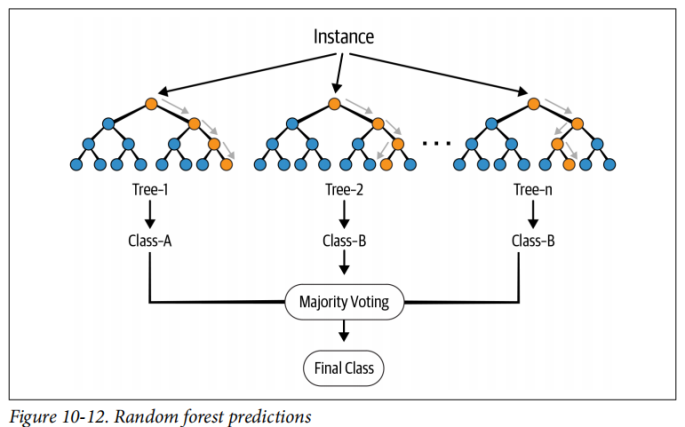k 折交叉验证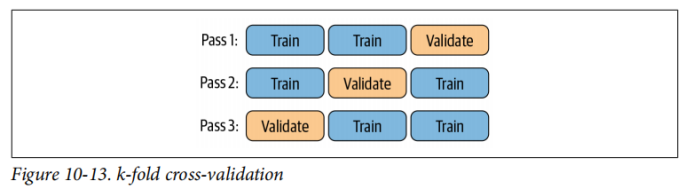1. 定义你要评估的 estimator。

2. 使用 ParamGridBuilder 指定要更改的超参数以及它们各自的有效值。

3. 定义一个 evaluator，以指定用于比较各种模型的指标。

4. 使用 CrossValidator 进行交叉验证，评估各个模型。

In Python

pipeline = Pipeline(stages = [stringIndexer, vecAssembler, rf])

// In Scala

val pipeline = new Pipeline()

.setStages(Array(stringIndexer, vecAssembler, rf))

（maxDepth = 2，numTrees = 10）

（maxDepth = 2，numTrees = 100）

（maxDepth = 4，numTrees = 10）

（maxDepth = 4，numTrees = 100）

（maxDepth = 6，numTrees = 10）

（maxDepth = 6，numTrees = 100）

In Python

from pyspark.ml.tuning import ParamGridBuilder

paramGrid = (ParamGridBuilder()

.build())

// In Scala

import org.apache.spark.ml.tuning.ParamGridBuilder

val paramGrid = new ParamGridBuilder()

.build()

In Python

evaluator = RegressionEvaluator(labelCol="price",

predictionCol="prediction",

metricName="rmse")

// In Scala

val evaluator = new RegressionEvaluator()

.setLabelCol("price")

.setPredictionCol("prediction")

.setMetricName("rmse")

In Python

from pyspark.ml.tuning import CrossValidator

cv = CrossValidator(estimator=pipeline,

evaluator=evaluator,

estimatorParamMaps=paramGrid,

numFolds=3,

seed=42)

cvModel = cv.fit(trainDF)

// In Scala

import org.apache.spark.ml.tuning.CrossValidator

val cv = new CrossValidator()

.setEstimator(pipeline)

.setEvaluator(evaluator)

.setEstimatorParamMaps(paramGrid)

.setNumFolds(3)

.setSeed(42)

val cvModel = cv.fit(trainDF)

Command took 1.07 minutes

In Python

list(zip(cvModel.getEstimatorParamMaps(), cvModel.avgMetrics))

// In Scala

cvModel.getEstimatorParamMaps.zip(cvModel.avgMetrics)

res1: Array[(org.apache.spark.ml.param.ParamMap, Double)] =

Array(({

rfr_a132fb1ab6c8-maxDepth: 2,

rfr_a132fb1ab6c8-numTrees: 10

},303.99522869739343), ({

rfr_a132fb1ab6c8-maxDepth: 2,

rfr_a132fb1ab6c8-numTrees: 100

},299.56501993529474), ({

rfr_a132fb1ab6c8-maxDepth: 4,

rfr_a132fb1ab6c8-numTrees: 10

},310.63687030886894), ({

rfr_a132fb1ab6c8-maxDepth: 4,

rfr_a132fb1ab6c8-numTrees: 100

},294.7369599168999), ({

rfr_a132fb1ab6c8-maxDepth: 6,

rfr_a132fb1ab6c8-numTrees: 10

},312.6678169109293), ({

rfr_a132fb1ab6c8-maxDepth: 6,

rfr_a132fb1ab6c8-numTrees: 100

},292.101039874209))

In Python

cvModel = cv.setParallelism(4).fit(trainDF)

// In Scala

val cvModel = cv.setParallelism(4).fit(trainDF)

Command took 31.45 seconds

In Python

cv = CrossValidator(estimator=rf,

evaluator=evaluator,

estimatorParamMaps=paramGrid,

numFolds=3,

parallelism=4,

seed=42)

pipeline = Pipeline(stages=[stringIndexer, vecAssembler, cv])

pipelineModel = pipeline.fit(trainDF)

// In Scala

val cv = new CrossValidator()

.setEstimator(rf)

.setEvaluator(evaluator)

.setEstimatorParamMaps(paramGrid)

.setNumFolds(3)

.setParallelism(4)

.setSeed(42)

val pipeline = new Pipeline()

.setStages(Array(stringIndexer, vecAssembler, cv))

val pipelineModel = pipeline.fit(trainDF)

Command took 26.21 seconds## 评论